# KSEEB Solutions for Class 10 Maths Chapter 15 Surface Areas and Volumes Ex 15.5

Students can Download Class 10 Maths Chapter 15 Surface Areas and Volumes Ex 15.5 Questions and Answers, Notes Pdf, KSEEB Solutions for Class 10 Maths helps you to revise the complete Karnataka State Board Syllabus and score more marks in your examinations.

## Karnataka State Syllabus Class 10 Maths Chapter 15 Surface Areas and Volumes Ex 15.5

Question 1.
A copper wire, 3 mm in diameter, is wound about a cylinder whose length is 12 cm, and diameter 10 cm, so as to cover the curved surface of the cylinder. Find the length and mass of the wire, assuming the density of copper to be 8.88 g per cm3.
Cylinder
Diameter = 10 cm,
radius = r = $$\frac{d}{2}=\frac{10}{2}$$ = 5cm
Length of wire one round of cylinder = circumference of base
= 2πr = 2π(5) = 10π cm
Cylindrical wire diameter = 3mm
= $$\frac{3}{10}$$ cm
length of cylinder = h = 12 cmTotal round = 40
Now, length of wire required in one round = circumference of base of cylinder
2πr = 2π × $$\frac{10}{2}$$
2πr = 10π cm
Hence in 40round the length of wire required
= 40 × 10π
= 400π cm
= $$\frac{400 \times 22}{7}$$ = 1257.14 cm
= 12.57 m.
therefore total length of wire 12.57 m
volume of wire = πr2h
= π × $$\left(\frac{0.3}{2}\right)^{2}$$ × 1257.14
88.898cm3
The mass of wire of 1 cm3 = 8.885
∴ the mass of wire of 88.898 cm3
= 8.88 × 88.898
= 798.413
Hence mass of wire = 798.419 and length of wire = 12.57 mQuestion 2.
A right triangle, whose sides are 3 cm and 4 cm (other than hypotenuse) is -made to revolve about its hypotenuse. Find the volume and surface area of the double cone so formed. (Choose value of π as found appropriate.)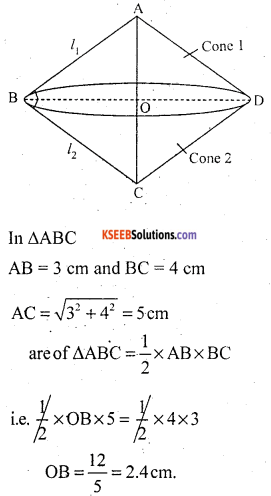Hence OB = radius of cone = 2.4 cm
Volume of double cone = volume of cone 1 + volume of cone 2.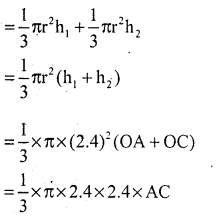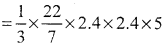= 30.14cm3
Volume of double cone = 30.14 cm3
Surface are of double cone
= CSA 1 + CSA 2Question 3.
A cistern, internally measuring 150 cm × 120 cm × no cm, has 129600 cm3 of water in it. Porous bricks are placed in the water until the cistern is full to the brim. Each brick absorbs one-seventeenth of its own volume of water. How many bricks can be put in without overflowing the water, each brick being 22.5 cm × 7.5 cm × 6.5 cm?
Volume of cistern =150 × 120 × 110
= 1980000 cm3
volume of water in cistern
= 129600 cm3
volume to filled in cistern
= 1980000 – 129600
= 1850400 cm3
volume of one brick = 22.5 × 7.5 × 6.5
= 1096.875 cm3
Let ‘N’ no of bricks can be placed into the cistern
Hence volume of ‘N’ no of bricks
= N × 1096.875 cm3
One bricks absorb water $$\frac{1}{17}$$ of his volume hence water absorb bv 1 brickHence total N no. of brick absorb waterVolume to be filled in tank + water absorbs by N no. of bricks = volume of N No of bricks.∴ therefore No. of bricks can be put in cistern without over flowing water.Question 4.
In one fortnight of a given month, there was a rainfall of 10 cm in a 5. river valley. If the area of the valley is 7280 km2, show that the total rainfall was approximately equivalent to the addition to the normal water of three rivers each 1072 km long, 75 m wide and 3 m deep.
For each of three rivers.
length (l) = 1072 km = 1072 × 1000 m
= 1072000 m.
Breadth = b = 75 m and Depth = h = 3 m.
volume of water in river = lbh
= 1072000 × 75 × 3
= 241200000 m3.
Volume of water in 3 rivers
= 3 × 241200000
= 723600000 m3.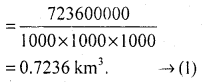Area of the valley = 7280 km2
Rainfall in the valleyVolume of rainfall = Area of the valley × height of rainfall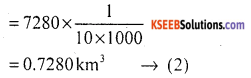From (1) and (2) total rainfall is approximately equivalent

Question 5.
An oil funnel made of tin sheet consists of a 10 cm long cylindrical portion attached to a frustum of a cone. If the total height is 22 cm, diameter of the cylindrical portion is 8 cm and the diameter of the top of the funnel is 18 cm, find the area of the tin sheet required to make the funnel (see Fig. 15.25).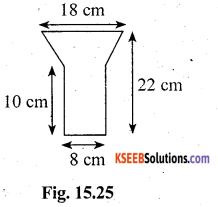r1 = $$\frac{18}{2}$$ = 9cm , r2 = $$\frac{8}{2}$$ = 4 cm
Height h of frustum = 22 – 10 = 12 cm.
Slant height of the frustum cone =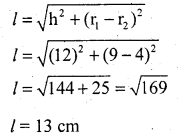l = 13cm
radius of cylindrical portion = $$\frac{8}{2}$$
= 4 cm.
Area of tin sheet required = CSA of cylinder + CSA of frustum portion
= 2πrh + π (r1 + r2) l
= 2π(4)(10) + π(9 + 4) 13
= 80π + π × 13 × 13 = 80π + 169π
= 249π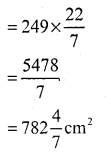error: Content is protected !!